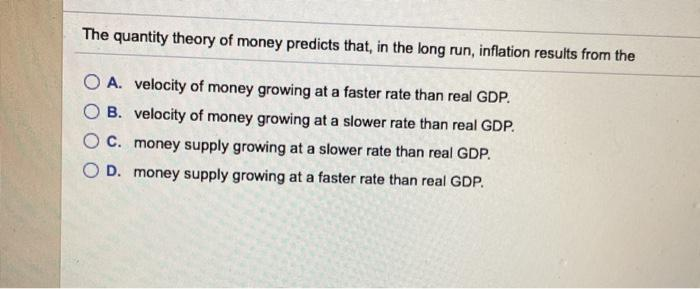# The quantity theory of money predicts that. in the long run. inflation results from theThe quantity theory of money predicts that, in the long run, inflation results from the O A. velocity of money growing at a faster rate than real GDP. B. velocity of money growing at a slower rate than real GDP. O c. money supply growing at a slower rate than real GDP. OD. money supply growing at a faster rate than real GDP.

D) Money supply growing at a faster rate then real GDP
The quantity theory of money predicts that in the long run
inflation result from the money supply growing at a faster rate
then real GDP.